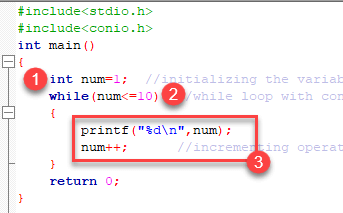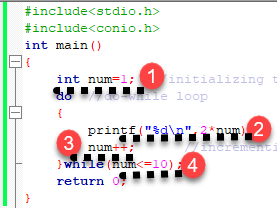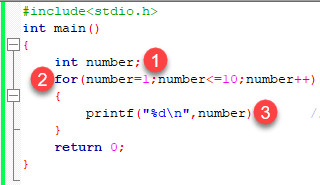# C Loops: For, While, Do While, Break, Continue with Example

## What are Loops?

In looping, a program executes the sequence of statements many times until the stated condition becomes false. A loop consists of two parts, a body of a loop and a control statement. The control statement is a combination of some conditions that direct the body of the loop to execute until the specified condition becomes false.

In this tutorial, you will learn-

## Types of Loops

Depending upon the position of a control statement in a program, a loop is classified into two types:

1. Entry controlled loop

2. Exit controlled loop

In an entry controlled loop, a condition is checked before executing the body of a loop. It is also called as a pre-checking loop.

In an exit controlled loop, a condition is checked after executing the body of a loop. It is also called as a post-checking loop.

The control conditions must be well defined and specified otherwise the loop will execute an infinite number of times. The loop that does not stop executing and processes the statements number of times is called as an infinite loop. An infinite loop is also called as an “Endless loop.” Following are some characteristics of an infinite loop:

1. No termination condition is specified.

2. The specified conditions never meet.

The specified condition determines whether to execute the loop body or not.

‘C’ programming language provides us with three types of loop constructs:

1. The while loop

2. The do-while loop

3. The for loop

## While Loop

A while loop is the most straightforward looping structure. The basic format of while loop is as follows:

```while (condition) {
statements;
}
```

It is an entry-controlled loop. In while loop, a condition is evaluated before processing a body of the loop. If a condition is true then and only then the body of a loop is executed. After the body of a loop is executed then control again goes back at the beginning, and the condition is checked if it is true, the same process is executed until the condition becomes false. Once the condition becomes false, the control goes out of the loop.

After exiting the loop, the control goes to the statements which are immediately after the loop. The body of a loop can contain more than one statement. If it contains only one statement, then the curly braces are not compulsory. It is a good practice though to use the curly braces even we have a single statement in the body.

In while loop, if the condition is not true, then the body of a loop will not be executed, not even once. It is different in do while loop which we will see shortly.

Following program illustrates a while loop:

```#include<stdio.h>
#include<conio.h>
int main()
{
int num=1;	//initializing the variable
while(num<=10)	//while loop with condition
{
printf("%d\n",num);
num++;		//incrementing operation
}
return 0;
}
```

Output:

```1
2
3
4
5
6
7
8
9
10
```

The above program illustrates the use of while loop. In the above program, we have printed series of numbers from 1 to 10 using a while loop.1. We have initialized a variable called num with value 1. We are going to print from 1 to 10 hence the variable is initialized with value 1. If you want to print from 0, then assign the value 0 during initialization.
2. In a while loop, we have provided a condition (num<=10), which means the loop will execute the body until the value of num becomes 10. After that, the loop will be terminated, and control will fall outside the loop.
3. In the body of a loop, we have a print function to print our number and an increment operation to increment the value per execution of a loop. An initial value of num is 1, after the execution, it will become 2, and during the next execution, it will become 3. This process will continue until the value becomes 10 and then it will print the series on console and terminate the loop.

\n is used for formatting purposes which means the value will be printed on a new line.

## Do-While loop

A do-while loop is similar to the while loop except that the condition is always executed after the body of a loop. It is also called an exit-controlled loop.

The basic format of while loop is as follows:

``` do {
statements
} while (expression);```

As we saw in a while loop, the body is executed if and only if the condition is true. In some cases, we have to execute a body of the loop at least once even if the condition is false. This type of operation can be achieved by using a do-while loop.

In the do-while loop, the body of a loop is always executed at least once. After the body is executed, then it checks the condition. If the condition is true, then it will again execute the body of a loop otherwise control is transferred out of the loop.

Similar to the while loop, once the control goes out of the loop the statements which are immediately after the loop is executed.

The critical difference between the while and do-while loop is that in while loop the while is written at the beginning. In do-while loop, the while condition is written at the end and terminates with a semi-colon (;)

The following program illustrates the working of a do-while loop:

We are going to print a table of number 2 using do while loop.

```#include<stdio.h>
#include<conio.h>
int main()
{
int num=1;	//initializing the variable
do	//do-while loop
{
printf("%d\n",2*num);
num++;		//incrementing operation
}while(num<=10);
return 0;
}
```

Output:

```2
4
6
8
10
12
14
16
18
20
```

In the above example, we have printed multiplication table of 2 using a do-while loop. Let’s see how the program was able to print the series.1. First, we have initialized a variable ‘num’ with value 1. Then we have written a do-while loop.
2. In a loop, we have a print function that will print the series by multiplying the value of num with 2.
3. After each increment, the value of num will increase by 1, and it will be printed on the screen.
4. Initially, the value of num is 1. In a body of a loop, the print function will be executed in this way: 2*num where num=1, then 2*1=2 hence the value two will be printed. This will go on until the value of num becomes 10. After that loop will be terminated and a statement which is immediately after the loop will be executed. In this case return 0.

## For loop

A for loop is a more efficient loop structure in ‘C’ programming. The general structure of for loop is as follows:

```for (initial value; condition; incrementation or decrementation )
{
statements;
}
```
• The initial value of the for loop is performed only once.
• The condition is a Boolean expression that tests and compares the counter to a fixed value after each iteration, stopping the for loop when false is returned.
• The incrementation/decrementation increases (or decreases) the counter by a set value.

Following program illustrates the use of a simple for loop:

```#include<stdio.h>
int main()
{
int number;
for(number=1;number<=10;number++)	//for loop to print 1-10 numbers
{
printf("%d\n",number);		//to print the number
}
return 0;
}
```

Output:

```1
2
3
4
5
6
7
8
9
10
```

The above program prints the number series from 1-10 using for loop.1. We have declared a variable of an int data type to store values.
2. In for loop, in the initialization part, we have assigned value 1 to the variable number. In the condition part, we have specified our condition and then the increment part.
3. In the body of a loop, we have a print function to print the numbers on a new line in the console. We have the value one stored in number, after the first iteration the value will be incremented, and it will become 2. Now the variable number has the value 2. The condition will be rechecked and since the condition is true loop will be executed, and it will print two on the screen. This loop will keep on executing until the value of the variable becomes 10. After that, the loop will be terminated, and a series of 1-10 will be printed on the screen.

In C, the for loop can have multiple expressions separated by commas in each part.

For example:

```for (x = 0, y = num; x < y; i++, y--) {
statements;
}
```

Also, we can skip the initial value expression, condition and/or increment by adding a semicolon.

For example:

```int i=0;
int max = 10;
for (; i < max; i++) {
printf("%d\n", i);
}
```

Notice that loops can also be nested where there is an outer loop and an inner loop. For each iteration of the outer loop, the inner loop repeats its entire cycle.

Consider the following example, that uses nested for loops output a multiplication table:

```#include <stdio.h>
int main() {
int i, j;
int table = 2;
int max = 5;
for (i = 1; i <= table; i++) { // outer loop
for (j = 0; j <= max; j++) { // inner loop
printf("%d x %d = %d\n", i, j, i*j);
}
printf("\n"); /* blank line between tables */
}}```

Output:

```1 x 0 = 0
1 x 1 = 1
1 x 2 = 2
1 x 3 = 3
1 x 4 = 4
1 x 5 = 5

2 x 0 = 0
2 x 1 = 2
2 x 2 = 4
2 x 3 = 6
2 x 4 = 8
2 x 5 = 10
```

The nesting of for loops can be done up-to any level. The nested loops should be adequately indented to make code readable. In some versions of ‘C,’ the nesting is limited up to 15 loops, but some provide more.

The nested loops are mostly used in array applications which we will see in further tutorials.

## Break Statement

The break statement is used mainly in in the switch statement. It is also useful for immediately stopping a loop.

We consider the following program which introduces a break to exit a while loop:

```#include <stdio.h>
int main() {
int num = 5;
while (num > 0) {
if (num == 3)
break;
printf("%d\n", num);
num--;
}}
```

Output:

```5
4
```

## Continue Statement

When you want to skip to the next iteration but remain in the loop, you should use the continue statement.

For example:

```#include <stdio.h>
int main() {
int nb = 7;
while (nb > 0) {
nb--;
if (nb == 5)
continue;
printf("%d\n", nb);
}}
```

Output:

```6
4
3
2
1
```

So, the value 5 is skipped.

## Which loop to Select?

Selection of a loop is always a tough task for a programmer, to select a loop do the following steps:

• Analyze the problem and check whether it requires a pre-test or a post-test loop.
• If pre-test is required, use a while or for a loop.
• If post-test is required, use a do-while loop.

Summary

• Looping is one of the key concepts on any programming language.
• It executes a block of statements number of times until the condition becomes false.
• Loops are of 2 types: entry-controlled and exit-controlled.
• ‘C’ programming provides us 1) while 2) do-while and 3) for loop.
• For and while loop is entry-controlled loops.
• Do-while is an exit-controlled loop.